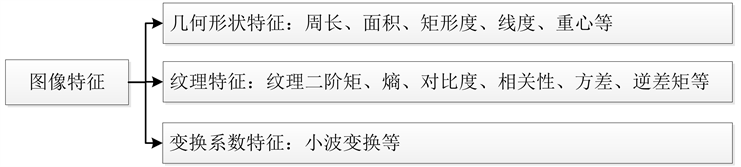﻿ 基于多特征值综合目标提取技术的注塑零件表面缺陷检测方法

# 基于多特征值综合目标提取技术的注塑零件表面缺陷检测方法Surface Defect Detection Method for Injection Parts Based on Multi-Feature Integrated Target Extraction Technology

Abstract: Aimed at the characteristics of surface defects of injection parts, a method of surface defect detection based on multi-feature integrated target extraction is proposed. Characteristics of surface defects of injection molded parts were analyzed. With target extraction technologies of features of geometry, texture and transform coefficients, 13 different characteristic parameters were extracted through the data dimensionality reduction and the surface defect characteristics were determined. Based on 13 characteristic values, class distance normal binary tree SVM was used as the classifier of surface defect for defect judgment. Experiment shows that the surface defect detection method can effectively extract the integrated targets, and then achieves accurate detection of the surface defects in injection parts.

1. 引言

2. 综合目标提取技术

2.1. 表面特征种类

2.2. 表面特征提取方法Figure 1. Types of image feature

2.2.1. 几何特征提取方法

1) 周长(l)：描述目标区域的周长。可以利用图像分割处理后生成的二值图像，对图像边界上像素个数的统计来获取周长特征值。

2) 面积(area)：描述目标区域的大小。对于此特征值的提取可以利用目标边界内像素个数来获取面积的特征值。假设待检测产品图像f(x,y)的大小为M × N，经过二值化处理，用逻辑1表示目标部分，则面积的计算公式为：

$area=\underset{x=1}{\overset{M}{\sum }}\underset{y=1}{\overset{N}{\sum }}f\left(x,y\right)$ (1)

3) 矩形度(rect)：表面缺陷含有毛边等涉及边界的缺陷，因此，可以用矩形度判别目标区域是否有缺陷。其计算式为：

$rect=\frac{area}{A}$ (2)

4) 线度(X)：反映单位边界长度所围成的目标区域面积的大小。其计算公式为：

$X=\frac{area}{l}$ (3)

2.2.2. 纹理特征提取方法

${P}_{d}=\left[\begin{array}{cccccc}{P}_{d}\left(0,0\right)& {P}_{d}\left(0,1\right)& \cdots & {P}_{d}\left(0,j\right)& \cdots & {P}_{d}\left(0,L-1\right)\\ {P}_{d}\left(1,0\right)& {P}_{d}\left(1,1\right)& \cdots & {P}_{d}\left(1,j\right)& \cdots & {P}_{d}\left(1,L-1\right)\\ ⋮& ⋮& & ⋮& & ⋮\\ {P}_{d}\left(i,0\right)& {P}_{d}\left(i,1\right)& \cdots & {P}_{d}\left(i,j\right)& \cdots & {P}_{d}\left(i,L-1\right)\\ ⋮& ⋮& & ⋮& & ⋮\\ {P}_{d}\left(L-1,0\right)& {P}_{d}\left(L-1,1\right)& \cdots & {P}_{d}\left(L-1,J\right)& \cdots & {P}_{d}\left(L-1,L-1\right)\end{array}\right]$

2.2.3. 变换系数特征提取方法

$e=\frac{1}{{M}^{2}}\underset{m,n=1}{\overset{M}{\sum }}x\left(m,n\right)$ (4)

$Energy=\frac{{\sum }_{m}{\sum }_{n}{x}^{2}\left(m,n\right)}{M×M}$ (5)

$Entropy=\frac{{\sum }_{m}{\sum }_{n}{x}^{2}\left(m,n\right)\mathrm{log}\left({x}^{2}\left(m,n\right)\right)}{M×M}$ (6)

3. 多特征值的确定

4. 检测实例Table 1. Selection of experimental eigenvaluesTable 2. Geometric feature parameters of defective image

5. 结论Table 3. Texture feature parameters of defective imageTable 4. Parameters of wavelet transform in defective image

 He, Z., Wang, Y., Yin, F., et al. (2016) Surface Defect Detection for High-Speed Rails Using an Inverse P-M Diffusion Model. Sensor Review, 36, 86-97.
https://doi.org/10.1108/SR-03-2015-0039

 周文举. 基于机器视觉的在线髙速检测与精确控制题研究及应用[D]. 上海: 上海大学, 2013: 14-16

 Liu, J. and Macgregor, J.F. (2005) Modeling and Optimization of Product Appearance: Application to Injection-Molded Plastic Panels. Industrial Engineering and Chemistry Research, 44, 4687-4696.
https://doi.org/10.1021/ie0492101

 Karimi, M.H. and Asemani, D. (2014) Surface Defect Detection in Tiling Industries Using Digital Image Processing Methods: Analysis and Evaluation. Isa Transactions, 53, 834-844.
https://doi.org/10.1016/j.isatra.2013.11.015

 Li, G., Shi, J., Luo, H., et al. (2013) A Computational Model of Vision Attention for Inspection of Surface Quality in Production Line. Machine Vision & Applications, 24, 835-844.
https://doi.org/10.1007/s00138-012-0429-1

 刘阳. 注塑制品质量参数在线检测建模与优化方法研究[D]. 沈阳: 东北大学, 2010: 2-4.

Top